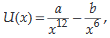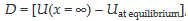# The potential energy function for the force between two atoms in a diatomic molecule is approximately given by  where a and b are constants...

The potential energy function for the force between two atoms in a diatomic molecule is approximately given bywhere a and b are constants and x is the distance between the atoms. What will be D, if the dissociation energy of the molecule isAnonymous User Physics 12 May, 2020 60 views